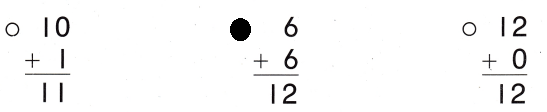Refer to our Texas Go Math Grade 1 Answer Key Pdf to score good marks in the exams. Test yourself by practicing the problems from Texas Go Math Grade 1 Lesson 6.2 Answer Key Add Doubles.

Essential Question
What are doubles facts?
Explanation:
Double Facts are additions in which a number is added to itself. For example, 1 + 1, 2 + 2 etc. Internalizing double facts are helpful building blocks in developing fluency in adding single digit numbers.

Explore
UseDraw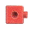to solve. Write the addition sentence.

___ + __ = ___
4 + 4 = 8
Sal used 8 to build the tower

For The Teacher

• Read the following problem. Sal built two towers. Each tower has 4 cubes. How many cubes does Sal use to build both towers?
Explanation:
4 + 4 = 8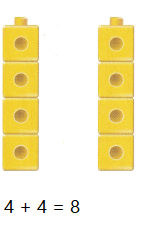Math Talk
Mathematical Processes
Describe how your model shows a doubles fact.
Explanation:
Double Facts are additions in which a number is added to itself.
For example, 1 + 1, 2 + 2 etc.

Model and Draw

Why are these doubles facts?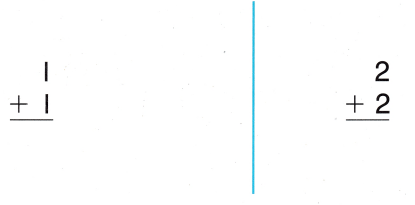Explanation: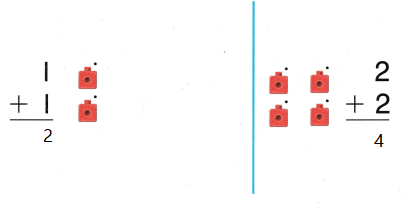Explanation:
The number is added to itself is called double fact
so, the above examples are double fact

Share and Show

UseDrawto show your work. Write the sum.

Question 1.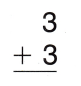Explanation:
With the help of Blocks
Written the sum of double facts

Question 2.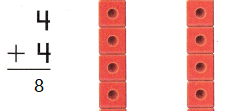Explanation:
With the help of Blocks
Written the sum of double facts
4 + 4 = 8

Question 3.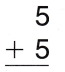Explanation:
With the help of Blocks
Written the sum of double facts
5 + 5 = 10

Question 4.Explanation:
With the help of Blocks
Written the sum of double facts
6 + 6 = 12

Problem Solving

UseDraw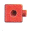to show your work.

Write the sum.

Question 5.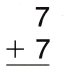Explanation:
With the help of Blocks
Written the sum of double facts
7 + 7 = 14

Question 6.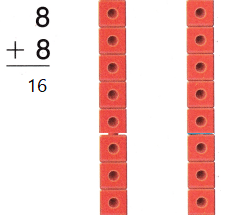Explanation:
With the help of Blocks
Written the sum of double facts
8 + 8 = 16

Write a doubles fact to solve.

Question 7.
H.O.T. There are 18 people at the party. Some are boys and some are girls. The number of boys is the same as the number of girls.
___ = __ + ___9 + 9 = 18
Explanation:
There are 18 people at the party.
Some are boys and some are girls.
8 boys and 8 girls
The number of boys is the same as the number of girls.

Question 8.
H.O.T. Multi-Step Mike got 1 point in the first game and 1 point in the second game. Emily got 10 points in the first game and 10 points in the second game. How many points did each child get?
Mike
___ + ___ = ___ points
Emily
___ + ___ = ___ points
Compare the number of points.
____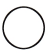____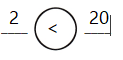Explanation:
Mike
1 + 1 = 2 points
Emily
10 + 10 = 20 points
Mike got 2 and Emily got 20

Question 9.
There are 5 friends standing by the flag. There are 5 friends running up the How many friends are there?
(A) 10
(B) 9
(C) 8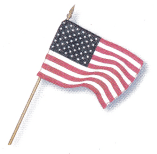Explanation:
5 + 5 = 10
The number which is added to itself is called a double fact
there are 10 friends.

Question 10.
Julian has 4 red cups. He has 4 blue cups. How many cups does Julian have?
(A) 7
(B) 8
(C) 9
Explanation:
The number which is added to itself is called a double fact
4 + 4 = 8
8 cups that Julian have

Question 11.
Reasoning Which doubles fact shows how many fish in all?
(A) 2 + 2 = 4
(B) 6 + 6 = 12
(C) 3 + 3 = 6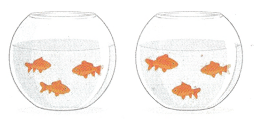Explanation:
The number which is added to itself is called a double fact
3 + 3 = 6

Question 12.
Texas Test Prep Which is a doubles fact?Explanation:
The number which is added to itself is called a double fact
so, 5 + 5 = 10 is a double fact.

Take Home Activity

• Have your child choose o number from 1 to 10, and use that number in a doubles fact. Repeat with other numbers.
Explanation:
Choose the number from 1 to 10
that is 5
5 + 5 = 10

### Texas Go Math Grade 1 Lesson 6.2 Homework and Practice Answer Key

Drawto solve. Write the sum.

Question 1.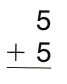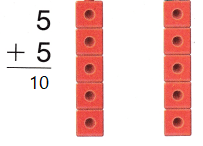Explanation:
With the help of Blocks
Written the sum of double facts
The number which is added to itself is called a double fact
5 + 5 = 10

Question 2.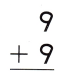Explanation:
With the help of Blocks
Written the sum of double facts
The number which is added to itself is called a double fact
9 + 9 = 18

Question 3.
Clara has 7 big fish. She has 7 small fish. Write the doubles fact that shows how many fish Clara has in all.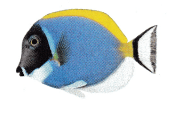___ + ___ = ____
7 + 7 = 14
Explanation:
The number which is added to itself is called a double fact
14 fish Clara has in all

Question 4.
The room has 2 big windows. It also has 2 small windows. How many windows are there?
___ + ___ = ____
2 + 2 = 4
Explanation:
4 windows are there in all
The number which is added to itself is called a double fact

Problem Solving

Write a doubles fact to solve.

Question 5.
There are 16 animals in the yard. Some are cats and some are dogs. The number of cats is the same as the number of dogs.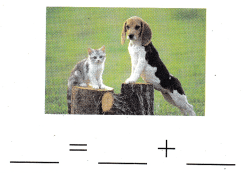8 + 8 = 16
Explanation:
cats are 8 and dogs are 8
so, 8 + 8 = 16

Texas Test Prep

Question 6.
There are 6 flowers in the front yard. There are 6 flowers in the back yard. How many flowers are there?
(A) 12
(B) 10
(C) 6
Explanation
The number which is added to itself is called a double fact
6 + 6 = 12
12 flowers are there

Question 7.
Which doubles fact shows how many eggs in all?
(A) 6 + 6 = 12
(B) 4 + 4 = 8
(C) 3 + 3 = 6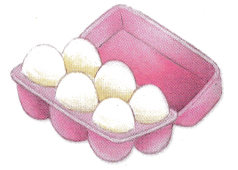Explanation:
The number which is added to itself is called a double fact
3 eggs arranged in 2 rows
3 + 3 = 6

Question 8.
Multi-Step Jan saved 4 pennies the first day and 4 pennies the second day. Clint saved 6 pennies the first day and 6 pennies the second day. Which statement compares the total number of pennies each child saved?
(A) 5 < 6 (B) 11 > 4
(C) 8 < 12
Explanation:
4 + 4 = 8
6 + 6 = 12
8 < 12 statement compares the total number of pennies each child saved

Question 9.
Which is a doubles fact?